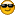###Author Topic: C++ display vector elements not working  (Read 523 times)

####modeezy23

• Single posting newcomer
•• Posts: 5##### C++ display vector elements not working
« on: April 19, 2020, 06:51:25 pm »
Hi all, I'm an amateur to programming and I'm just practicing. How come my my vector does not display the previous results? Thank you in advance. Also, if any of you have any advice or input on how I can improve on my coding please let me know.

#include <iostream>
#include <vector>

using namespace std;

//This function will subtract 2 integers and return the result.
int subtract(){
int num1;
int num2;
cout << "Enter 2 integers to subtract: ";
cin >> num1;
cin >> num2;

int result = num1 - num2;
return result;
}

//This function will add 2 integers and return the result.

int num1;
int num2;
cout << "Enter 2 integers to add: ";
cin >> num1;
cin >> num2;

int result = num1 + num2;
return result;
}

//This funtion will display the menu.
int num;

cout << " Add two numbers. " << endl;
cout << " Subtract two numbers. " << endl;
cout << " Display previous results." << endl;
cout << " EXIT" << endl;
cout << "PLEASE ENTER A NUMBER TO EXECUTE: " << endl;
cin >> num;

return num;
}

//This function will ask user if they want to choose again.
cout << "Would you like to choose again?: ";
char choice;
cin >> choice;
return choice;
}

//This is the main function.
int main(){

vector<int> vect;

// int variables are declared/initialized.
int choice;
int result;

//This will be executed if option 1 is selected.
if(num == 1){
cout << "The answer is: " << result << endl;
vect.push_back(result);
if(choice == 'Y'){
main();
}
else{
cout << "GOODBYE!";
}
}
//This will be executed if option 2 is selected.
else if(num == 2){
result = subtract();
cout << "The answer is: " << result;
vect.push_back(result);
if(choice == 'Y'){
main();
}
else{
cout << "GOODBYE!";
}
}
//Executed if option 3 is selected.
else if(num == 3){
cout << "The previous results are: " << endl;
for(int i = 0; i < vect.size(); i++){
cout << vect.at(i);
}
}

//This will be executed if option 4 is selected.
else if(num == 4){
cout << "GOODBYE!";
}

return 0;
}

####BlueHazzard

• Developer
• Lives here!
•• Posts: 2850##### Re: C++ display vector elements not working
« Reply #1 on: April 20, 2020, 08:44:24 am »
Hi,
1) This is not a general programming forum. So question like "Why does my code not work" are not allowed here.
2) If you post code, please use code tags (The # symbol in the new forum post editor)
3) If you have problems and post a question to any forum, you have to provide useful information, your post has none... To answer your post (and not loose tons of time by the guy who has to answer) Always add the following information to your posts:
1) Operating system
2) Compiler, compiler version, software version (codeblocks version)
3) What have you done
4) What should your program do
5) What it actually does
6) Do you have any idea why it does not work?
7) Have you tried already something? What have you tried?

Things like
Quote
How come my my vector does not display the previous results?
should more be like
Quote
I expect my program to output the content of the vector variable, because if i enter the menu 3) my program should work trough loop in line number XX.
The expected output is "1, 2, 3" but i actually get "". What could be the cause of this?  I have used the debugger but XXXXUse the debugger to search for your error. You can find plenty youtube videos. Search for "codeblocks debugger".

I hope my list helps you to post better questions the next time...
Have a nice day